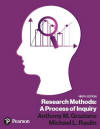﻿ Other Nonparametric StatisticsGraziano & Raulin
Research Methods (9th edition)

## Other Nonparametric Statistics

At one point, nonparametric tests were common in psychology, and they represented a significant portion of the statistical coverage at both an undergraduate and graduate level. That is less true now for three reasons.

The first is that computer simulation studies (called Monte Carlo studies) have shown that the violation of statistical assumptions have less impact on parametric statistical procedures than once thought. In fact, when sample sizes are reasonably large and approximately equal, you can violate almost any statistical assumption without significantly distorting most statistical procedures.

The second reason that nonparametric statistics are used less frequently is that there are often better dependent measures available than were once used. Over decades of work, the most widely used measures in a given area have been refined so that they produce the kinds of distributions that are handled well by traditional statistical procedures.

The final reason that nonparametric statistics are used less frequently today is that alternative strategies have been developed that work at least as well in reducing statistical problems. The most common of these alternative strategies is to transform the data. Transformations are mathematical changes in which the same mathematical function is applied to every data point in the study. These transformations can change the shape of distributions, thus reducing the violations of statistical assumptions.

Nevertheless, there are times when the most reasonable statistical procedure is a nonparametric test. These tend to be used in a few areas of psychology in which it is impossible to find dependent measures that produce the kind of distributions necessary for parametric statistical tests and areas in which it is common to be working with relatively small samples. We describe some of the more commonly used tests below.

A wide variety of nonparametric statistics have been developed for use in the social sciences. The best single resource for this statistics is Siegel & Castellan (1988). We will briefly describe several tests, without going into the a discussion of how they are computed.

• Mann-Whitney U-test: The Mann-Whitney U-test is used to compare two independent groups on ordered data (ranks).

• Wilcoxon Test: The Wilcoxon test is the nonparametric equivalent to the correlated t-test. It compares two correlated groups (either within-subjects or matched-subjects designs), using ranks instead of scores.

• Sign Test for Matched Samples:  This is another nonparametric procedure for testing two correlated samples. In the Wilcoxon, the scores were converted to ranks. Here the scores are converted to directions only. So the test looks at the number of differences that are positive versus the number of differences that are negative.

• The Median Test for Two or More Independent Samples: As the name implies, this procedure compares the medians of groups instead of the means of the groups.

### Reference

Siegel, S., & Castellan, N. J. (1988). Nonparametric statistics for the behavioral sciences (2nd ed.). New York: McGraw-Hill.